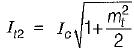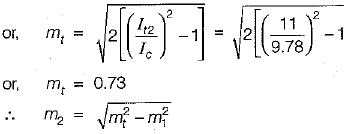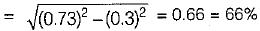Courses

# Amplitude Modulation

## 10 Questions MCQ Test Topicwise Question Bank for Electronics Engineering | Amplitude Modulation

Description
This mock test of Amplitude Modulation for GATE helps you for every GATE entrance exam. This contains 10 Multiple Choice Questions for GATE Amplitude Modulation (mcq) to study with solutions a complete question bank. The solved questions answers in this Amplitude Modulation quiz give you a good mix of easy questions and tough questions. GATE students definitely take this Amplitude Modulation exercise for a better result in the exam. You can find other Amplitude Modulation extra questions, long questions & short questions for GATE on EduRev as well by searching above.
QUESTION: 1

### The carrier amplitude after AM varies between 4 volts and 1 volt. The depth of modulation is

Solution:

We know that,
Modulation index;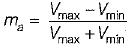Given, Vmax = 4 volt and Vmin  = 1 volt
∴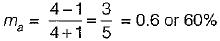QUESTION: 2

### A sinusoidal carrier has an amplitude of 10 V and frequency 30 kHz. It is amplitude modulated by a sinusoidal voltage of amplitude 3 V and frequency 1 kHz. The spectrum of AM wave will be represented as

Solution:

Given,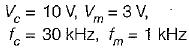∴ Modulation index,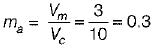The sideband frequencies are as under: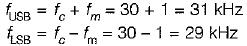Amplitude of each sideband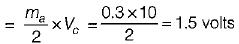Hence, the spectrum of AM wave will be as shown in figure below.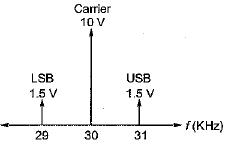QUESTION: 3

### Assertion (A): The principle of envelope detection of an AM signal is based on multiplying the incoming signal with the locally generated synchronous carrier and pass the product through a LPF. Reason (R): An envelope detector produces an output signal that follows the envelope of the input AM signal exactly.

Solution:

The principle of envelope detection of an AM signal is based on rectifying the incoming signal and passing it through a LPF. Hence, assertion is false. Assertion is the principle of synchronous detection.

QUESTION: 4

Consider the following statements associated with the synchronous detection method for detection/demodulation of AM signals:
1. The circuit complexity is very less.
2. It introduces frequency and phase errors only.
3. it does not require any locally generated synchronous carrier.
4. It can be used for DSB-SC, SSB and VSB but not for DSB-FC.
Which of the statements given above is/are correct?

Solution:

• The circuit complexity of synchronous detection is very high. Hence, statement-1 is not correct.
• Statement-2 is correct.
• Synchronous detection require a locally generated synchronous carrier. Hence, statement-3 is not correct.
• Statement-4 is correct because synchronous detection is used for DSB-SC, SSB and VSB while envelope detection is used for DSB-FC only.

QUESTION: 5

Assertion (A): in amplitude modulation, the higher percentage of modulation is preferred.
Reason (R): The modulation index of more than 100% can be achieved without compromising the envelope distortion.
(a) Both A and R are true and R is the correct explanation of A.
(b) Both A and R are true but R is not the correct explanation of A.
(c) A is true but R is false.
(d) A is false but R is true.

Solution:

In amplitude modulation, the higher percentage of modulation is preferred because the higher value of percentage of modulation results into a strong and more intelligible received signal which is less likely to get contaminated or lost in the noise. A modulation index of more than 100% results into over modulation which introduces envelope distortion. Hence, assertion is true but reason is false.

QUESTION: 6

The types of distortions which can occur in the envelope detector output is/are

Solution:
QUESTION: 7

A 400 Watts carrier is modulated to a depth of 75 percent. Assuming the modulating signal to a sinusoidal one, the total power in the amplitude-modulated wave is

Solution:

Given,
Pc = 400 Watt, ma = 0.75
The total power in the amplitude modulated wave is: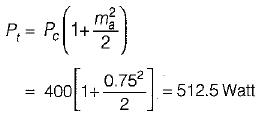QUESTION: 8

The modulation index of the low level emitter modulator is 0.7, voltage gain without modulating signal is AQ = 100, carrier frequency fc = 1 MHz. The amplitude of carrier is 10 mV. If the modulating frequency is 1 kHz, the range of voltage gain is

Solution:

We have: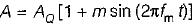or,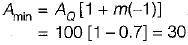and,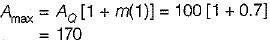Hence, the range of A = 30 to 170

QUESTION: 9

The antenna current of an AM transmitter is 8 A if only the carrier is sent, but it increases to 8.93 A if the carrier is modulated by a single sinusoidal wave. What is the antenna current when the percent of modulation is 80%?

Solution:

Given, Ic = 8 a, ma = 0.8
We know that, antenna current is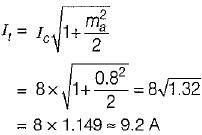QUESTION: 10

The antenna current of an AM transmitter is 10 A when it is modulated to depth of 30% by an audio signal. It increases to 11 A when another signal modulates the carrier signal. The modulation index due to second signal is

Solution:

Given,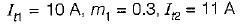We know that, the overall modulation index is: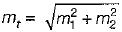or,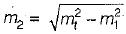Now,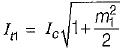or,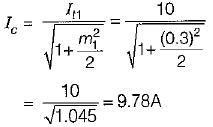After modulating with the second signal, we have